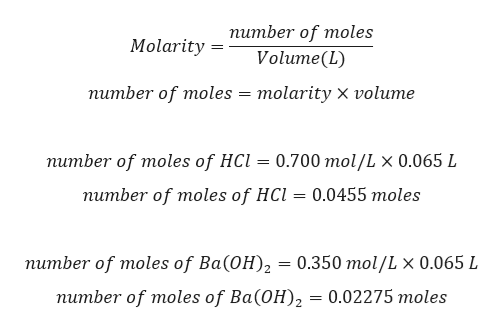# In a constant‑pressure calorimeter, 65.0 mL of 0.350 M Ba(OH)2 was added to 65.0 mL of 0.700 M HCl.The reaction caused the temperature of the solution to rise from 22.90 ∘C to 27.7 ∘C. If the solution has the same density and specific heat as water (1.00 g/mL and 4.184J/g⋅°C,) respectively), what is Δ? for this reaction (per mole H2O produced)? Assume that the total volume is the sum of the individual volumes.Δ?=             kJ/mol H2O

Question
In a constant‑pressure calorimeter, 65.0 mL of 0.350 M Ba(OH)2 was added to 65.0 mL of 0.700 M HCl.

The reaction caused the temperature of the solution to rise from 22.90 ∘C to 27.7 ∘C. If the solution has the same density and specific heat as water (1.00 g/mL and 4.184J/g⋅°C,) respectively), what is Δ? for this reaction (per mole H2O produced)? Assume that the total volume is the sum of the individual volumes.

Δ?=             kJ/mol H2O
check_circleExpert Solution
Step 1

Given:

65.0 mL of 0.350 M Ba(OH)2

65.0 mL of 0.700 M HCl.

Ba(OH)2 + 2 HCl → BaCl2 + 2 H2O

Step 2

Calculation for number of moles of HCl and Ba(OH)2:help_outlineImage Transcriptioncloseпитber of moles Molarity Volume(L) питber of moles 3D тolaritу х volume питber of moles of HCl — 0.700 тol/L x 0.065 L питber of moles of HCl 3D 0.0455 тoles питber of moles of Ba(ОH), — 0.350 тol/L x 0.065 L питber of moles of Ba(ОH), — 0.02275 тoles fullscreen
Step 3

Identifying limiting reactant,

Number of moles HCl = 0.0455 moles/2 = 0.02275 moles

Number of moles Ba(OH)2 = 0...

### Want to see the full answer?

See Solution

#### Want to see this answer and more?

Solutions are written by subject experts who are available 24/7. Questions are typically answered within 1 hour*

See Solution
*Response times may vary by subject and question
Tagged in

### Chemical Thermodynamics